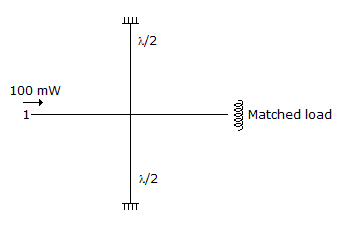# Electronics and Communication Engineering - Microwave Communication

Exercise : Microwave Communication - Section 8
1.
Consider the following statements, SWR of a transmission line is infinite when the load is
1. a short circuit
2. a complex impedance
3. an open circuit
4. a pure reactance
Which of the above statements are correct?
1 and 3 only
1, 2, 3
2, 3, 4
1, 2, 4
Explanation:
No answer description is available. Let's discuss.

2.
In a reflex klystron the output frequency is determined primarily by resonant frequency of cavity.
True
False
Explanation:
No answer description is available. Let's discuss.

3.
The arrangement in the given figure is a hybid T. If 100 mW fed at port 1, the power reflected back at port 1 is0
25 mW
50 mW
100 mW
Explanation:
No answer description is available. Let's discuss.

4.
In TE10 mode the largest electric field in a rectangular wave guide, occurs
along centre line of broad wall (i.e. x = a/2)
along the broad wall (i.e. x = 0 and x = a)
either (a) or (b)
neither (a) nor (b)